# Evaluating Expressions with Two Variables - Khan Academy

##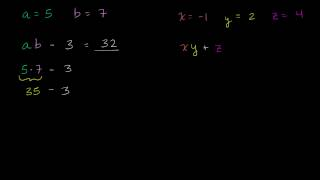By Khan Academy

Sal Khan shows the viewer how to evaluate an expression with two variables.# Evaluating expressions with variables word problems

##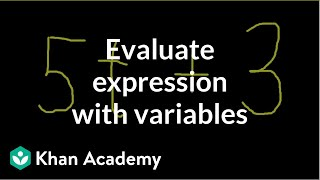By Khan Academy

Learn how to evaluate an expression with variables using a technique called substitution (or plugging in).# Evaluating Expressions Involving Absolute Value - Example 1

##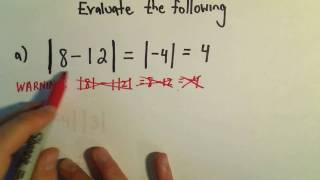By PatrickJMT

This video explains how to evaluate expressions involving absolute value. The examples include a few very basic problems involving simplifications with absolute value.# Evaluate expressions

##By MathPlanetVideos

Evaluate the following expression for x = 2, y = 5 and z = 4 4x+(7−z)−6y# Evaluating Expressions

##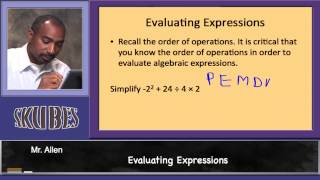By Skubes Ed

Interpret expressions that represent a quantity in terms of its context. Interpret parts of an expression, such as terms, factors, and coefficients.# Evaluating expressions with variables word problems

##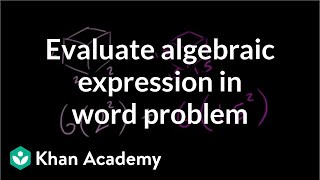By Khan Academy

In this example of evaluating expressions, we're dusting off some geometry. On top of that, it's a word problem. We're seeing how different concepts in math are layered on top of each to create more interesting and complex problems to solve.# Evaluating expressions with variables word problems

##By Khan Academy

Learn how to evaluate an expression with variables using a technique called substitution (or plugging in).# Evaluating expressions with variables word problems

##By Khan Academy

Learn how to evaluate an expression with variables using a technique called substitution (or plugging in).# Evaluating expressions with variables word problems

##By Khan Academy

Learn how to evaluate an expression with variables using a technique called substitution (or plugging in).# Evaluating Expressions Part 1 - Khan Academy

##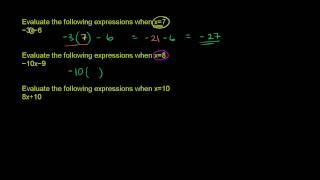By Khan Academy

This is a very brief video clip about Evaluating Expressions Part 1.# Ex: Evaluate an Expression with Rational Exponents Using Radicals

##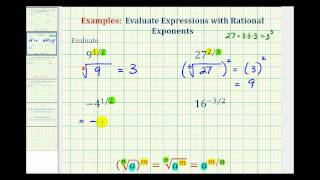By Mathispower4u

This video provides examples of how to evaluate an expressions with rational exponents by writing the expression in radical form and then simplifying.# Evaluating Expressions with Parentheses

##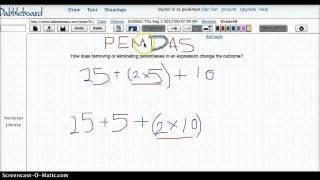By KFreimuth83

This Screencast was completed using Dabbleboard and shows how changing removing or eliminating parentheses with similar numbers can change the outcome. The instructor reviews the order of operations as she works the same problem in two different ways based on placement of parentheses.# Evaluating Expressions with Parentheses and Braces and Brackets

##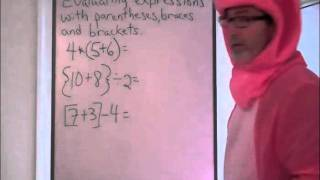By HoodaMathTutorials

The instructor dressed as a pig shows how to solve expressions using parentheses braces and brackets. He explains that when these symbols are used they mean to "do me first" in order of operations.# Evaluating expressions with variables word problems

##By Khan Academy

In this example of evaluating expressions, we're dusting off some geometry. On top of that, it's a word problem. We're seeing how different concepts in math are layered on top of each to create more interesting and complex problems to solve.# Evaluating expressions with variables word problems

##By Khan Academy

In this example of evaluating expressions, we're dusting off some geometry. On top of that, it's a word problem. We're seeing how different concepts in math are layered on top of each to create more interesting and complex problems to solve.# Evaluating expressions with variables word problems

##By Khan Academy

In this example of evaluating expressions, we're dusting off some geometry. On top of that, it's a word problem. We're seeing how different concepts in math are layered on top of each to create more interesting and complex problems to solve.# Evaluating expressions with variables word problems

##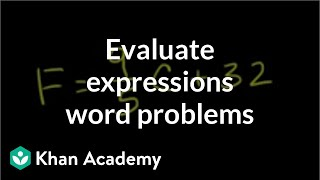By Khan Academy

In this example we have a formula for converting Celsius temperature to Fahrenheit. Let's substitute the variable with a value (Celsius temp) to get the degrees in Fahrenheit. Great problem to practice with us!# Order of Operations examples

##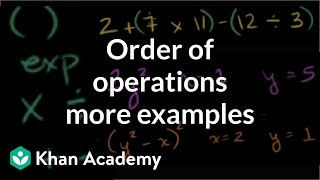By Khan Academy

Evaluating expressions using order of operations# Absolute value

##By MathPlanetVideos

Evaluate the following expression |x|+11,x=−9# Properties of a Rhombus | MathHelp.com

##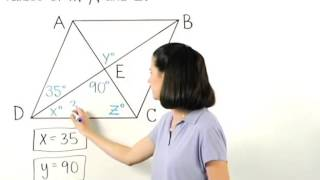By MathHelp.com

This lesson covers evaluating expressions. Students learn to evaluate expressions by substituting the given integers in for the variables in the given expression, then simplifying. Students also learn to use parentheses when substituting.